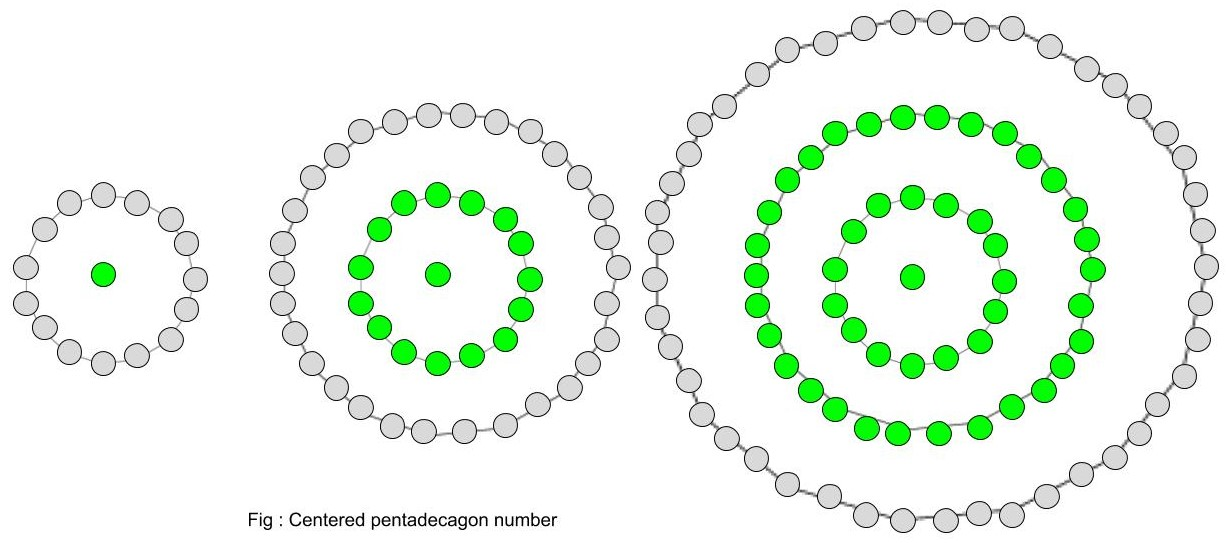# Centered Pentadecagonal Number

• Last Updated : 20 May, 2022

Given a number n, find the nth Centered Pentadecagonal Number .
A Centered Pentadecagonal Number represents a dot in the centre and other dots surrounding it in successive pentadecagonal(15-sided polygon) layers.Examples :

```Input :  2
Output : 16

Input : 8
Output : 421```

n-th term of Centered pentadecagonal number:-

``

Below is the basic implementation of the above idea.

## C++

 `// C++ Program to find``// nth centered``// pentadecagonal  number``#include ``using` `namespace` `std;` `// centered pentadecagonal function``int` `center_pentadecagonal_num(``long` `int` `n)``{``    ``// Formula to calculate nth``    ``// centered pentadecagonal number``    ``return` `(15 * n * n - 15 * n + 2) / 2;``}` `// Driver Code``int` `main()``{``    ``long` `int` `n = 3;``    ``cout << n << ``"th number : "``             ``<< center_pentadecagonal_num(n);``    ``cout << endl;``    ``n = 10;``    ``cout << n << ``"th number : "``             ``<< center_pentadecagonal_num(n);` `    ``return` `0;``}`

## C

 `// C Program to find``// nth centered``// pentadecagonal  number``#include ` `// centered pentadecagonal function``int` `center_pentadecagonal_num(``long` `int` `n)``{``    ``// Formula to calculate nth``    ``// centered pentadecagonal number``    ``return` `(15 * n * n - 15 * n + 2) / 2;``}` `// Driver Code``int` `main()``{``    ``long` `int` `n = 3;``    ``printf``(``"%ldth number : %d\n"``,n,center_pentadecagonal_num(n));` `    ``n = 10;``    ``printf``(``"%ldth number : %d\n"``,n,center_pentadecagonal_num(n));` `    ``return` `0;``}` `// This code is contributed by kothavvsaakash.`

## Java

 `// Java Program to find nth centered``// pentadecagonal number` `import` `java.io.*;` `class` `GFG {``    ` `    ``// centered pentadecagonal function``    ``static` `long` `center_pentadecagonal_num(``long` `n)``    ``{``    ` `        ``// Formula to calculate nth``        ``// centered pentadecagonal number``        ``return` `(``15` `* n * n - ``15` `* n + ``2``) / ``2``;``    ``}``    ` `    ``// Driver Code``    ``public` `static` `void` `main (String[] args)``    ``{``    ` `        ``long` `n = ``3``;``        ``System.out.print(n + ``"th number : "``);``        ``System.out.println(``                  ``center_pentadecagonal_num(n));``        ` `        ``n = ``10``;``        ``System.out.print( n + ``"th number : "``);``        ``System.out.println(``                 ``center_pentadecagonal_num(n));``    ``}``}` `// This code is contributed by ajit.`

## Python3

 `# Program to find nth``#centered pentadecagonal number` `def` `center_pentadecagonal_num(n) :``    ` `    ``# Formula to calculate nth``    ``# centered pentadecagonal number``    ``return` `(``15` `*` `n ``*` `n ``-` `15` `*` `n ``+` `2``) ``/``/` `2` `# Driver Code``if` `__name__ ``=``=` `'__main__'` `:``        ` `    ``n ``=` `3``    ``print``(n,``"rd number : "``,``                ``center_pentadecagonal_num(n))``    ``n ``=` `10``    ``print``(n,``"th number : "``,``                 ``center_pentadecagonal_num(n))``                 `  `# This code is contributed  by m_kit`

## C#

 `// C# Program to find``// nth centered``// pentadecagonal number``using` `System;` `class` `GFG``{``    ` `    ``// centered``    ``// pentadecagonal function``    ``static` `long` `center_pentadecagonal_num(``long` `n)``    ``{``    ` `        ``// Formula to calculate``        ``// nth centered``        ``// pentadecagonal number``        ``return` `(15 * n * n -``                ``15 * n + 2) / 2;``    ``}``    ` `    ``// Driver Code``    ``static` `public` `void` `Main ()``    ``{``        ``long` `n = 3;``        ``Console.Write(n + ``"th number : "``);``        ``Console.WriteLine(``                ``center_pentadecagonal_num(n));``        ` `        ``n = 10;``        ``Console.Write( n + ``"th number : "``);``        ``Console.WriteLine(``                ``center_pentadecagonal_num(n));``    ``}``}` `// This code is contributed by ajit.`

## PHP

 ``

## Javascript

 ``

Output :

```3th number : 46
10th number : 676```

Time Complexity: O(1)
Auxiliary Space: O(1)

My Personal Notes arrow_drop_up